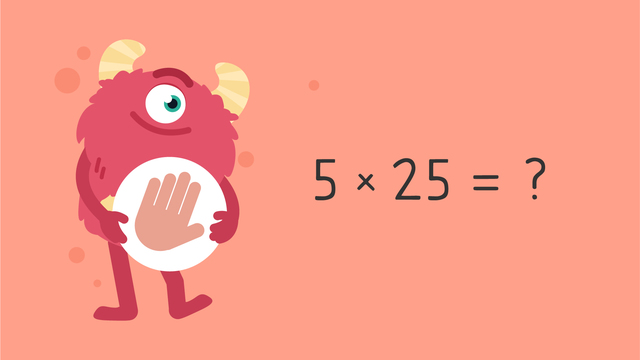# Grams and Kilograms (Word Problems) — Let's Practice!Rating

Ø 3.9 / 15 ratings
The authorsTeam Digital
Grams and Kilograms (Word Problems) — Let's Practice!
CCSS.MATH.CONTENT.3.MD.A.2

## Basics on the topicGrams and Kilograms (Word Problems) — Let's Practice!

Today we are practicing grams and kilograms word problems with Razzi! This video contains examples to help you further practice and grow confident in this topic.

### TranscriptGrams and Kilograms (Word Problems) — Let's Practice!

Razzi says get these items ready, because today we're going to practice grams and kilograms - word problems. It's time to begin! Solve this word problem. Josiah has five bouncy balls. The balls weigh twenty-five grams each. What is the total weight of all the balls? Pause the video to work on the problem and press play when you are ready to see the solution! To solve, we need to calculate five multiplied by twenty-five, which is one hundred twenty-five. Add the unit of measurement. Did you also get one hundred twenty-five grams? Let's tackle the next word problem! There is a total of twenty-four kilograms of sand. If all the sand is shared equally into eight bags, how many kilograms of sand are in each bag? Pause the video to work on the problem, and press play when you are ready to see the solution! To solve, we need to calculate twenty-four divided by eight, which is three. Add the unit of measurement. Did you also get three kilograms? Let's tackle the final word problem! A pineapple weighs two hundred fifty grams, and a mango weighs one hundred seventy-seven grams. What is the total weight of the fruits? Pause the video to work on the problem, and press play when you are ready to see the solution! To solve, we need to calculate two hundred fifty plus one hundred-seventy seven, which is four hundred twenty-seven. Add the unit of measurement. Did you also get four hundred twenty-seven grams? Razzi had so much fun practicing with you today! See you next time!

## Grams and Kilograms (Word Problems) — Let's Practice! exercise

Would you like to apply the knowledge you’ve learned? You can review and practice it with the tasks for the video Grams and Kilograms (Word Problems) — Let's Practice!.
• ### What is the total mass?

Hints

What is the unit that is being used in the question? Be sure that this is also the unit in the answer.

The question says in total, this means that the two amounts must be added.

Solution

75 g + 220 g = 295 g

• ### Pip's suitcase.

Hints

When Pip is finding the total of more than one item, that means the items need to be added together,

If Pip is removing an amount, then you need to complete a subtraction equation.

Solution

The shorts and the book = 1,035 g or 1.035 kg

• the shorts weigh (430 g) and the book weighs (605 g)
• 430 + 605 = 1,035
• 1,035 g is equivalent to 1.035 kg
The shorts and the book plus the hat = 1,250 g.
• the shorts weigh (430 g), the book weighs (605 g) and the hat weighs (215 g)
• 430 + 605 + 215 = 1,250 g.

The weight when the book is removed is 645 g.

• 1,250 - 605 = 645 g.

• ### What do they weigh?

Hints

Since there are three ducks and we want to find the weight of one, we must divide the total amount by the number of ducks.

What is 1,800 ÷ 3?

Solution

Each duck weighs 600 g

• 1,800 ÷ 3 = 600 g

Hints

The original recipe makes 30 cookies, 60 cookies is double this, so you will need to double the ingredients.

10 cookies is one third of 30. This means that you need to find one third of the ingredients, or divide by 3.

Solution

A recipe to bake 30 cookies requires 160 g sugar and 210 g flour. The total mass of the dry ingredients is 370 g.

• 160 + 210 = 370 g
To make 60 cookies, the recipe would need 740 g of flour and sugar in total.
• 370 x 2 = 740 g
If you want to make fewer cookies, and only need a recipe for 10, then you should use 70 g of flour.
• 210 ÷ 3 = 70 g

• ### Baking cakes.

Hints

We are finding 7 groups of 10, so we need to multiply 7 by 10.

What is 7 times 10? We can skip count by 10 7 times to solve.

Solution

There are 7 cupcakes and each cupcake has 10 g of sugar.

• 7 x 10 = 70 g
• We would also get 70 g if we calculated 10 x 7.
• ### What do the kittens weigh?

Hints

Remember that there are 1,000 g in 1 kg, so 2 kg is the same as 2,000 g.

We are sharing a total amount between a smaller number, so we need to divide.

Count how many kittens there are altogether.

Solution

When these kittens were born, they weighed 2.8 kg in total. That means that each kitten weighed 400 g. We know this because we can convert 2.8 kg into 2,800 g, then divide this by the number of kittens, which is 7.